# Lissajous Figures – C Program

In this post I will show you how to calculate and plot the Lissajous Curves using C and Gnuplot.
We will use the following information:$x=\sin\theta$$y=A\sin(n\theta + \delta)$
We will create a program that calculates the values of the x and y for various$\theta$ values and for different n and store these values in a txt file. Then just plot it using Gnuplot.
We will create two functions called ‘x’ and ‘y’, that contain the definition of x and y respectively.

### PROGRAM:

/************************************
********LISSAJOUS FIGURES **********
************************************/
#include<stdio.h>
#include<math.h>
double x(double theta){
return sin(theta);
}
double y(double theta, double A,double n, double delta){
return A*sin(n*theta+delta);
}
main(){
double theta;
FILE *fp=NULL;
double A,n,delta;
fp=fopen("lissajous.txt","w");
/*Loop to calculate and store data-points*/
for(theta=0;theta<=4*M_PI;theta=theta+0.01){
/*Change A, n or delta here*/
A=1;
n=3;
delta=M_PI/4;
fprintf(fp,"%lf\t%lf\n",x(theta),y(theta,A,n,delta));
}
}


When you run the above C, it will generate a file called ‘lissajous.txt’ which would contain 2 columns of data-points.
The first column contains the ‘x’ values and the next one is for ‘y’ values.
These can be easily plotted using Gnuplot by using the following commands:

### GnuPlot Command

plot 'lissajous.txt' w l

### OUTPUT

For different values of$\delta ,A ; n$ the output looks as follows:$A=1 ; n=1 ; \delta=\pi/4$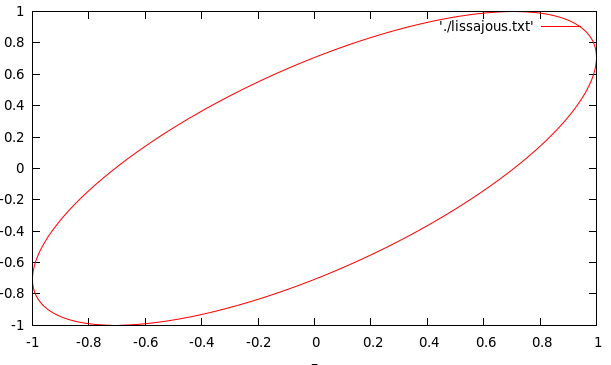$A=1 ; n=2 ; \delta =\pi/4$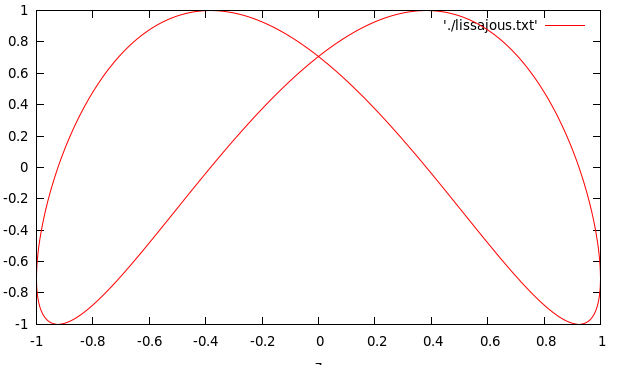$A=1; n=2.5 ; \delta =\pi/4$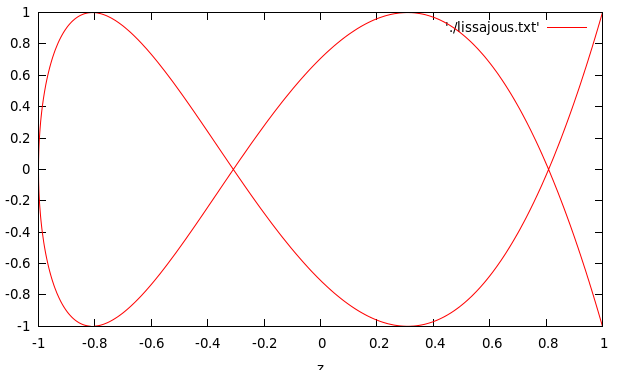$A=1; n=2 ; \delta =\pi/2$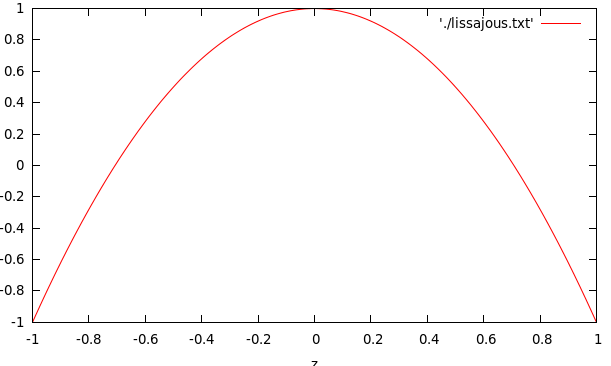$A=1 ; n=2; \delta = \pi$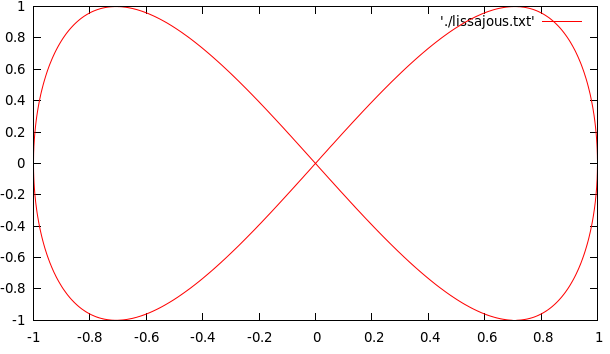$A=2 ; n=2; \delta = \pi$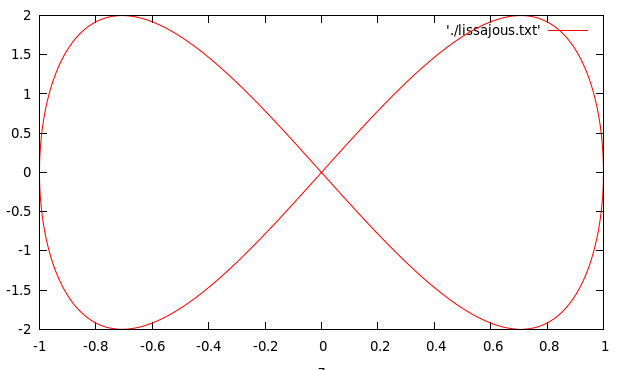### Resources: